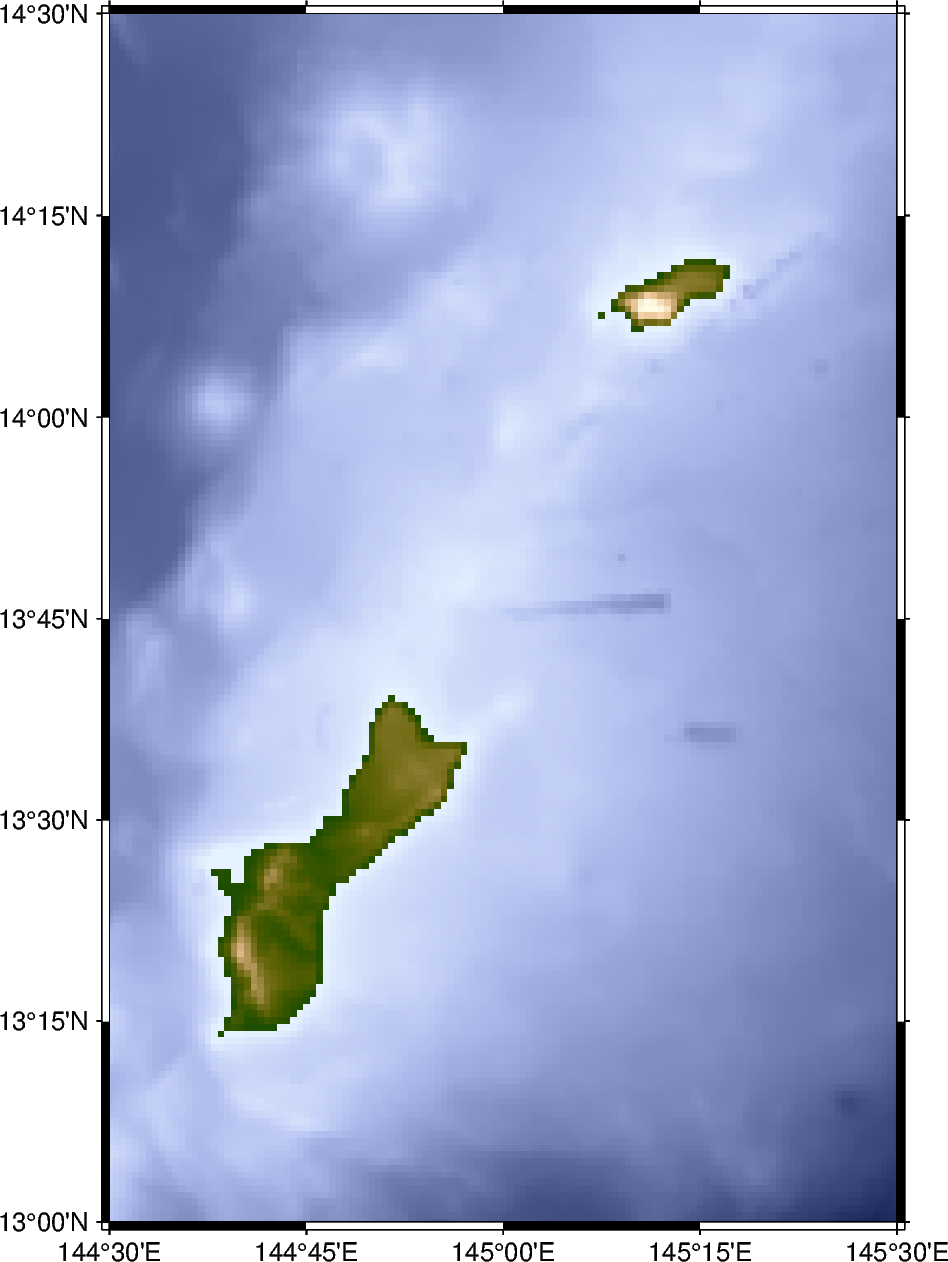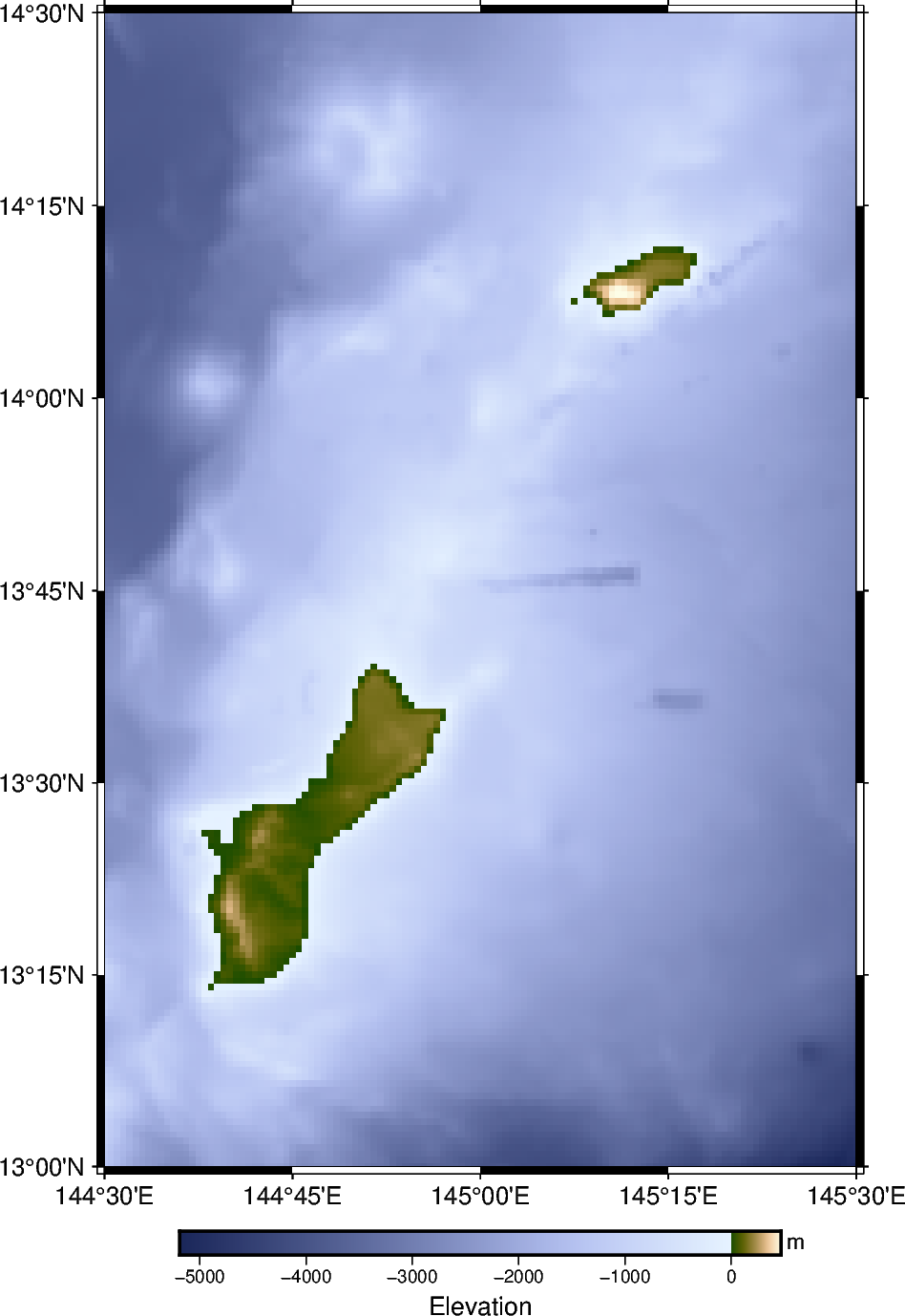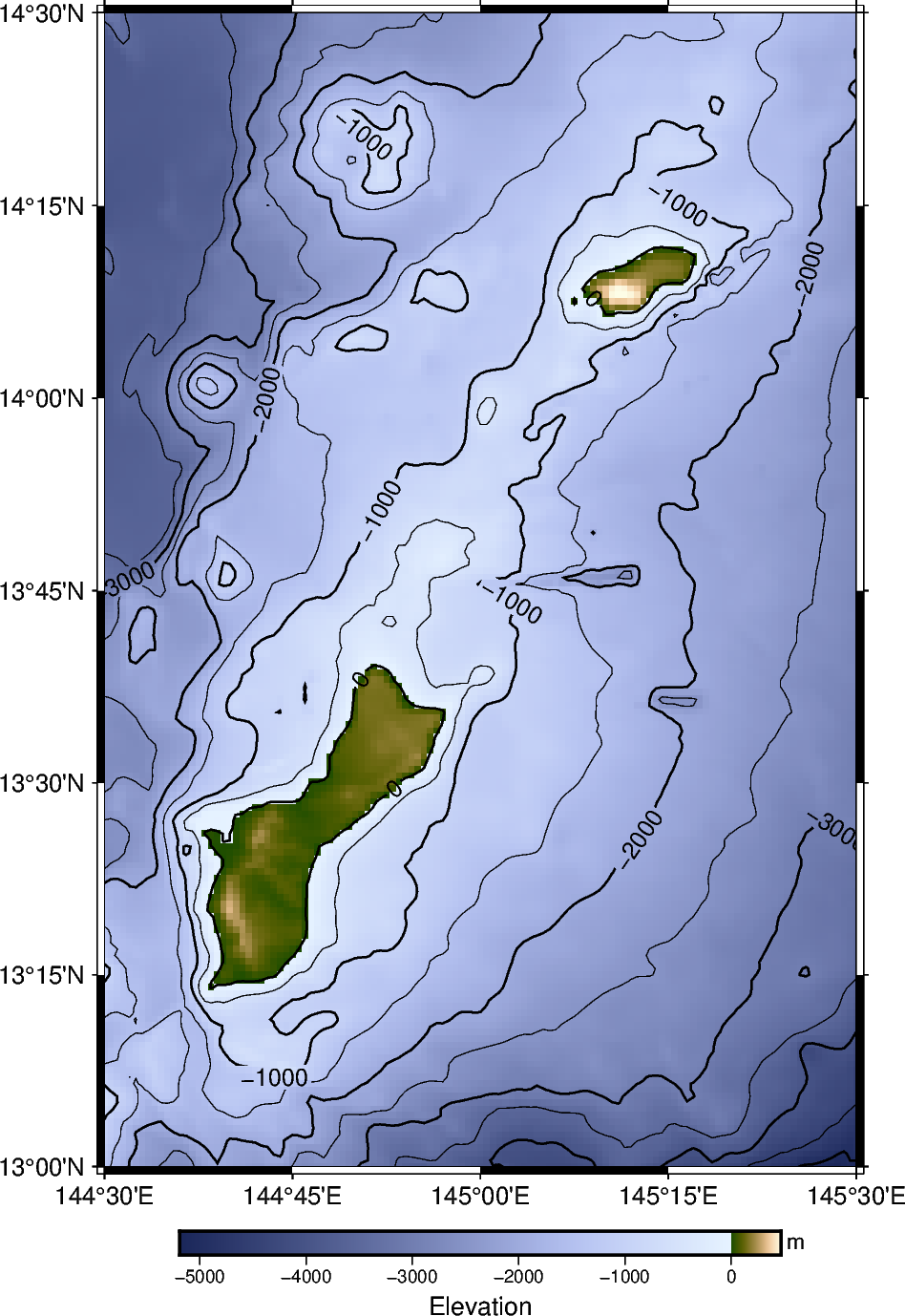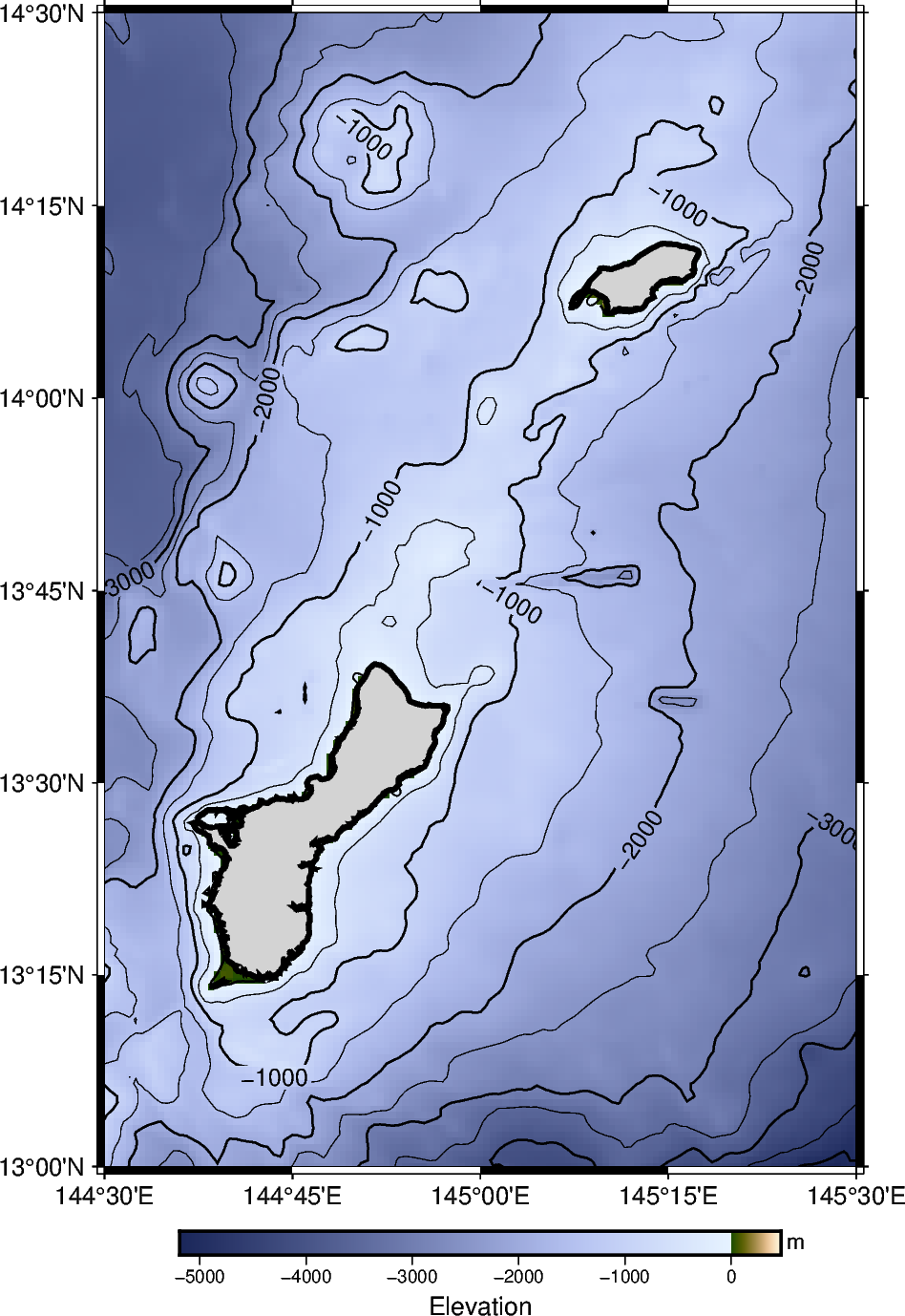# 2. Create a contour map

This tutorial page covers the basics of creating a figure of Earth relief, using a remote dataset hosted by GMT, using the method `pygmt.datasets.load_earth_relief`. It will use `pygmt.Figure.grdimage`, `pygmt.Figure.grdcontour`, `pygmt.Figure.colorbar`, and `pygmt.Figure.coast` methods for plotting.

```import pygmt
```

The first step is to use `pygmt.datasets.load_earth_relief`. The `resolution` parameter sets the resolution of the remote grid file, which will affect the resolution of the plot made later in the tutorial. The `registration` parameter determines the grid registration.

This grid region covers the islands of Guam and Rota in the western Pacific Ocean.

```grid = pygmt.datasets.load_earth_relief(
resolution="30s", region=[144.5, 145.5, 13, 14.5], registration="gridline"
)
```

## Plotting Earth relief

To plot Earth relief data, the method `pygmt.Figure.grdimage` can be used to plot a color-coded figure to display the topography and bathymetry in the grid file. The `grid` parameter accepts the input grid, which in this case is the remote file downloaded in the previous step. If the `region` parameter is not set, the region boundaries of the input grid are used.

The `cmap` parameter sets the color palette table (CPT) used for portraying Earth relief. The `pygmt.Figure.grdimage` method uses the input grid to apply Earth relief values to a specific color within the CPT. In this case, the CPT used is “oleron”; a full list of CPTs can be found at https://docs.generic-mapping-tools.org/latest/cookbook/cpts.html.

```fig = pygmt.Figure()
fig.grdimage(grid=grid, frame="a", projection="M10c", cmap="oleron")
fig.show()
```To show how the plotted colors relate to the Earth relief, a colorbar can be added using the `pygmt.Figure.colorbar` method.

To control the labels on the colorbar, a list is passed to the `frame` parameter. The value beginning with “a” sets the interval for annotation on the colorbar, in this case every 1,000 meters. To set the label for an axis on the colorbar, the value begins with either “x+l” (x-axis) or “y+l” (y-axis), followed by the intended label.

By default, the CPT for the colorbar is the same as the one set in `pygmt.Figure.grdimage`.

```fig = pygmt.Figure()
fig.grdimage(grid=grid, frame="a", projection="M10c", cmap="oleron")
fig.colorbar(frame=["a1000", "x+lElevation", "y+lm"])
fig.show()
```To add contour lines to the color coded figured, the `pygmt.Figure.grdcontour` method is used. The `frame` and `projection` are already set using `pygmt.Figure.grdimage` and are not needed again. However, the same input for `grid` (in this case, the variable named “grid”) must be input again. The `interval` parameter sets the spacing between lines (in this case, 500 meters), and the `annotation` parameter draws darker lines that are annotated with the elevation or bathymetry.

```fig = pygmt.Figure()
fig.grdimage(grid=grid, frame="a", projection="M10c", cmap="oleron")
fig.grdcontour(grid=grid, interval=500, annotation=1000)
fig.colorbar(frame=["a1000", "x+lElevation (m)"])
fig.show()
```## Color in land

To make it clear where the islands are located, the `pygmt.Figure.coast` method can be used to color in the land. The `land` is colored in as “lightgray”, and the `shorelines` parameters draws a border around the islands.

```fig = pygmt.Figure()
fig.grdimage(grid=grid, frame="a", projection="M10c", cmap="oleron")
fig.grdcontour(grid=grid, interval=500, annotation=1000)
fig.coast(shorelines="2p", land="lightgray")
fig.colorbar(frame=["a1000", "x+lElevation (m)"])
fig.show()
```This is the end of the second tutorial. Here are some additional exercises for the concepts that were discussed:

1. Change the resolution of the grid file to either “01m” (1 arc-minute, a lower resolution) or “15s” (15 arc-seconds, a higher resolution). Note that higher resolution grids will have larger file sizes. Available resolutions can be found here.

2. Create a contour map of the area around Mt. Rainier. A suggestion for the `region` would be `[-122, -121, 46.5, 47.5]`. Adjust the `pygmt.Figure.grdcontour` and `pygmt.Figure.colorbar` settings as needed to make the figure look good.

3. Create a contour map of São Miguel Island in the Azores; a suggested `region` is `[-26, -25, 37.5, 38]`. Instead of coloring in `land`, set `water` to “lightblue” to only display Earth relief information for the land.

4. Try other CPTs, such as “SCM/fes” or “geo”.

Total running time of the script: ( 0 minutes 3.011 seconds)

Gallery generated by Sphinx-Gallery